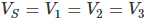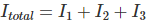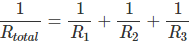# Voltage in Parallel Circuits (Sources, Formula & How To Add)

## What are Voltages in Parallel?

A parallel circuit or parallel connection refers to when two or more electrical devices are linked together in a side by side like arrangement within a circuit. In this connection, every device is located in its own distinct branch. Voltage (i.e. a potential difference) is the reason that current passes through a closed circuit. This article will discuss in detail voltages in a parallel circuit.

The multiple branch lines in a circuit mean there are several pathways for the charge to move to the external circuit. When a charge reaches a node or branching location, it makes a selection as to which branch should it pass through on its ride to reach back to the low potential terminal.

Let us consider a closed circuit of a voltage source and a resistor. In this circuit, the current will flow through the single pathway available. Next, in the same circuit, we add two further resistors in parallel with the first resistor.

It results in multiple pathways for the current to pass through rather than a single pathway to reach the low potential terminal. So, with the increase in the number of branches, the overall resistance decreases and it is obvious that the current in the circuit increases.

That is, the entire current will be the sum total of the different currents through the three resistors. Here in the parallel circuit, we can see that there are at most two sets of electrically common points. That is; A and H, B and G, C and F, D, and E in the circuit shown below. Voltage, which is measured across the common points for all time, should be equal.

In the above two figures, first shows the close circuit with a voltage source and a single resistor. The second one is the parallel circuit of 3 resistors and a voltage source.

The voltage in this circuit is the same for each and every three branches and it is also the same as the voltage of the source. Formulalicaly, that is:The total current in this given parallel circuit is represented by Itotal. The formula for this is given as.The total or effective resistance of this given parallel circuit is described in the formula below.Thus, we can conclude that by adding additional branches in a particular parallel circuit, the total current will get increased and the circuit gets overloaded.

When we want to connect two bulbs to a single battery, there are two options for us. Either it can be connected in an array or in parallel. If we connect it in an array, the two bulbs will be in the single and same conducting path between the two terminals of the battery. The problems with this connection are the following

• We cannot turn on or operate one bulb.
• Both the bulbs will be dim since they are using the same source.
• If there is a fault in one bulb, then the whole circuit will be affected.

Next, if we are connecting the two bulbs side by side or in parallel, the two bulbs will get the full voltage of the battery. So the result will be the following advantages.

• Both bulbs get the full amount of voltage in the battery.
• We can operate two bulbs separately.
• Both the bulbs will be bright when turned on.
• If there is a fault in one bulb, it can be removed or repaired. Thus, the whole circuit will not be affected.

## Parallel Circuits in Home

All our appliances at home are connected in parallel with each other. That is why we can operate every appliance separately without affecting others. For example, we can turn on the washing machine without turning on microwaves or television as well.

We know that in our house, the electrical cables comprises three wires- live, neutral and earth. For this moment we are ignoring the earth and simply concentrate on a live wire and a neutral wire.

The voltage is present across the live and neutral wire which is connected ultimately to a power plant. Each and every socket in our house is linked to these live and neutral. When we plug in a metal pin of an appliance into this socket, it creates an electrical connection with this socket.

Every appliance possesses its own connection among the live and neutral wire. So, when we switch on the device, the whole voltage will be present across it and it can be operated separately.

## Application of Voltage in Parallel

The applications of voltages in parallel include:

Want To Learn Faster? 🎓
Get electrical articles delivered to your inbox every week.
No credit card required—it’s 100% free.